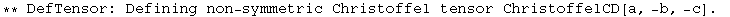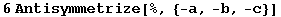6.3. General Bianchi identities

Let us now check the Bianchi identities for general covariant derivatives with torsion. Note that these identities are not directly encoded in xTensor`, but can be easily computed.

Define a covariant derivative with torsion. Note the special symmetry properties of the defined tensors:

In:=This is the general form of the first Bianchi identity:

In:=Out=In:=Out=The computation can be performed by transforming the derivative CD and its associated tensors into PD and Christoffel tensors:

In:=Out=In:=Out=In:=Out=In:=Out=This is the general form of the second Bianchi identity:

In:=Out=In:=Out=The computation proceeds along the same lines:

In:=Out=In:=Out=In:=Out=In:=Out=In:=Out=Clean up:

In:=Created by Mathematica  (May 16, 2008)